# balred

Model order reduction

## Syntax

``[rsys,info] = balred(sys,order)``
``[~,info] = balred(sys)``
``[___] = balred(___,opts)``
``balred(sys)``

## Description

example

````[rsys,info] = balred(sys,order)` computes a reduced-order approximation `rsys` of the LTI model `sys`. The desired order (number of states) is specified by `order`. You can try multiple orders at once by setting `order` to a vector of integers, in which case `rsys` is an array of reduced models. `balred` also returns a structure `info` with additional information like the Hankel singular values (HSV), error bound, regularization level and the Cholesky factors of the gramians.```

example

````[~,info] = balred(sys)` returns the structure `info` without computing the reduced-order model. You can use this information to select the reduced order `order` based on your desired fidelity. NoteWhen performance is a concern, avoid computing the Hankel singular values twice by using the information obtained from the above syntax to select the desired model order and then use `rsys = balred(sys,order,info)` to compute the reduced-order model. ```

example

````[___] = balred(___,opts)` computes the reduced model using the options set `opts` that you specify using `balredOptions` (Control System Toolbox). You can specify additional options for eliminating states, using absolute vs. relative error control, emphasizing certain time or frequency bands, and separating the stable and unstable modes. See `balredOptions` (Control System Toolbox) to create and configure the option set `opts`.```

example

````balred(sys)` displays the Hankel singular values and approximation error on a plot. Use `hsvplot` (Control System Toolbox) to customize this plot.```

## Examples

collapse all

For this example, use the Hankel singular value plot to select suitable order and compute the reduced-order model.

For this instance, generate a random discrete-time state-space model with 40 states.

```rng(0) sys = drss(40);```

Plot the Hankel singular values using `balred`.

`balred(sys)`For this example, select order of `16` since it is the first order with an absolute error less than `1e-4`. In general, you select the order based on the desired absolute or relative fidelity. Then, compute the reduced-order model.

`rsys = balred(sys,16);`

Verify the absolute error by plotting the singular value response using `sigma`.

`sigma(sys,sys-rsys)`Observe from the plot that the error, represented by the red curve, is below `-80 dB` (`1e-4`).

For this example, consider a random continuous-time state-space model with 65 states.

```rng(0) sys = rss(65); size(sys)```
```State-space model with 1 outputs, 1 inputs, and 65 states. ```

Visualize the Hankel singular values on a plot.

`balred(sys)`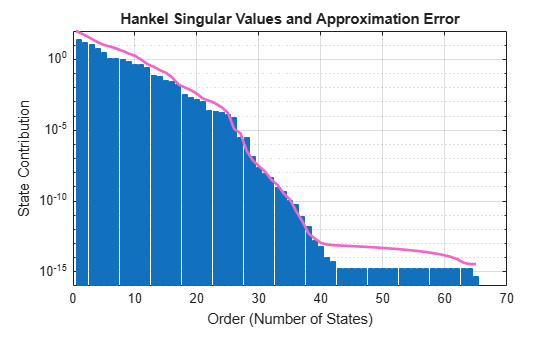For this instance, compute reduced-order models with 25, 30 and 35 states.

```order = [25,30,35]; rsys = balred(sys,order); size(rsys)```
```3x1 array of state-space models. Each model has 1 outputs, 1 inputs, and between 25 and 35 states. ```

Compute a reduced-order approximation of the system given by:

`$G\left(s\right)=\frac{\left(s+0.5\right)\left(s+1.1\right)\left(s+2.9\right)}{\left(s+{10}^{-6}\right)\left(s+1\right)\left(s+2\right)\left(s+3\right)}.$`

Create the model.

`sys = zpk([-0.5 -1.1 -2.9],[-1e-6 -2 -1 -3],1);`

Exclude the pole at $s=1{0}^{-6}$ from the stable term of the stable/unstable decomposition. To do so, set the `Offset` option of `balredOptions` to a value larger than the pole you want to exclude.

`opts = balredOptions('Offset',0.001,'StateProjection','Truncate');`

Visualize the Hankel singular values (HSV) and the approximation error.

`balred(sys,opts)`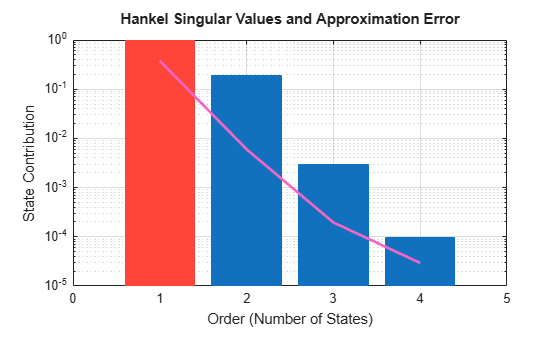Observe that the first HSV is red which indicates that it is associated with an unstable mode.

Now, compute a second-order approximation with the specified options.

```[rsys,info] = balred(sys,2,opts); rsys```
```rsys = 0.99113 (s+0.5235) ------------------- (s+1e-06) (s+1.952) Continuous-time zero/pole/gain model. ```

Notice that the pole at `-1e-6` appears unchanged in the reduced model `rsys`.

Compare the responses of the original and reduced-order models.

`bodeplot(sys,rsys,'r--')`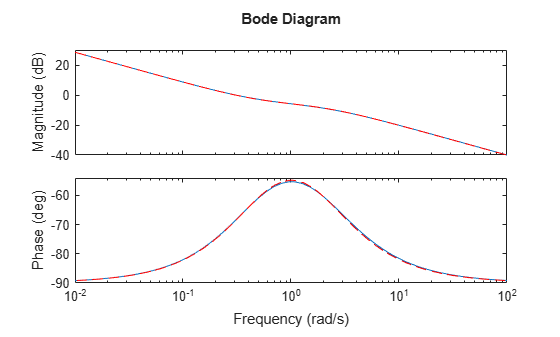Observe that the bode response of the original model and the reduced-order model nearly match.

Reduce a high-order model with a focus on the dynamics in a particular frequency range.

Load a model and examine its frequency response.

```load('highOrderModel.mat','G') bodeplot(G)````G` is a 48th-order model with several large peak regions around 5.2 rad/s, 13.5 rad/s, and 24.5 rad/s, and smaller peaks scattered across many frequencies. Suppose that for your application you are only interested in the dynamics near the second large peak, between 10 rad/s and 22 rad/s. Focus the model reduction on the region of interest to obtain a good match with a low-order approximation. Use `balredOptions` (Control System Toolbox) to specify the frequency interval for `balred`.

```bopt = balredOptions('StateProjection','Truncate','FreqIntervals',[10,22]); GLim10 = balred(G,10,bopt); GLim18 = balred(G,18,bopt);```

Examine the frequency responses of the reduced-order models. Also, examine the difference between those responses and the original response (the absolute error).

```subplot(2,1,1); bodemag(G,GLim10,GLim18,logspace(0.5,1.5,100)); title('Bode Magnitude Plot') legend('Original','Order 10','Order 18'); subplot(2,1,2); bodemag(G-GLim10,G-GLim18,logspace(0.5,1.5,100)); title('Absolute Error Plot') legend('Order 10','Order 18');```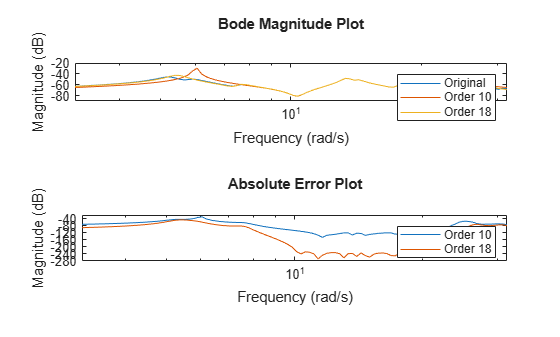With the frequency-limited energy computation, even the 10th-order approximation is quite good in the region of interest.

For this example, consider the SISO state-space model `cdrom` with 120 states. You can use absolute or relative error control when approximating models with `balred`. This example compares the two approaches when applied to a 120-state model of a portable CD player device `crdom` [1,2].

Load the CD player model `cdrom`.

```load cdromData.mat cdrom size(cdrom)```
```State-space model with 1 outputs, 1 inputs, and 120 states. ```

To compare results with absolute vs. relative error control, create one option set for each approach.

```opt_abs = balredOptions('ErrorBound','absolute','StateProjection','truncate'); opt_rel = balredOptions('ErrorBound','relative','StateProjection','truncate');```

Compute reduced-order models of order 15 with both approaches.

```rsys_abs = balred(cdrom,15,opt_abs); rsys_rel = balred(cdrom,15,opt_rel); size(rsys_abs)```
```State-space model with 1 outputs, 1 inputs, and 15 states. ```
`size(rsys_rel)`
```State-space model with 1 outputs, 1 inputs, and 15 states. ```

Plot the Bode response of the original model along with the absolute-error and relative-error reduced models.

```bo = bodeoptions; bo.PhaseMatching = 'on'; bodeplot(cdrom,'b.',rsys_abs,'r',rsys_rel,'g',bo) legend('Original (120 states)','Absolute Error (15 states)','Relative Error (15 states)')```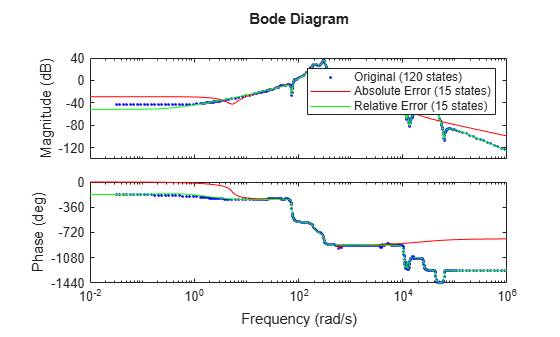Observe that the Bode response of:

• The relative-error reduced model `rsys_rel` nearly matches the response of the original model `sys` across the complete frequency range.

• The absolute-error reduced model `rsys_abs` matches the response of the original model `sys` only in areas with the most gain.

References

1. Benchmark Examples for Model Reduction, Subroutine Library in Systems and Control Theory (SLICOT). The CDROM data set is reproduced with permission, see BSD3-license for details.

2. A.Varga, “On stochastic balancing related model reduction”, Proceedings of the 39th IEEE Conference on Decision and Control (Cat. No.00CH37187), Sydney, NSW, 2000, pp. 2385-2390 vol.3, doi: 10.1109/CDC.2000.914156.

## Input Arguments

collapse all

Dynamic system, specified as a SISO or MIMO dynamic system model (Control System Toolbox). Dynamic systems that you can use can be continuous-time or discrete-time numeric LTI models, such as `tf`, `zpk` (Control System Toolbox), or `ss` (Control System Toolbox)models.

When `sys` has unstable poles, `balred` decomposes `sys` to its stable and unstable parts and only the stable part is approximated. Use `balredOptions` (Control System Toolbox) to specify additional options for the stable/unstable decomposition.

`balred` does not support frequency response data models, uncertain and generalized state-space models, PID models or sparse model objects.

Desired number of states, specified as an integer or a vector of integers. You can try multiple orders at once by setting `order` to a vector of integers, in which case `rys` is returned as an array of reduced models.

You can also use the Hankel singular values and error bound information to select the reduced-model order based on the desired model fidelity.

Additional options for model reduction, specified as an options set. You can specify additional options for eliminating states, using absolute vs. relative error control, emphasizing certain time or frequency bands, and separating the stable and unstable modes.

See `balredOptions` (Control System Toolbox) to create and configure the option set `opts`.

## Output Arguments

collapse all

Reduced-order model, returned as a dynamic system model or an array of dynamic system models.

Additional information about the LTI model, returned as a structure with the following fields:

• `HSV` — Hankel singular values (state contributions to the input/output behavior). In state coordinates that equalize the input-to-state and state-to-output energy transfers, the Hankel singular values measure the contribution of each state to the input/output behavior. Hankel singular values are to model order what singular values are to matrix rank. In particular, small Hankel singular values signal states that can be discarded to simplify the model.

• `ErrorBound` — Bound on absolute or relative approximation error. `info.ErrorBound(J+1)` bounds the error for order J.

• `Regularization` — Regularization level ⍴ (for relative error only). Here, `sys` is replaced by `[sys,⍴*I]` or `[sys;⍴*I]` that ensures a well-defined relative error at all frequencies.

• `Rr`, `Ro` — Cholesky factors of gramians.

## Algorithms

1. `balred` first decomposes G into its stable and unstable parts:

`$G={G}_{s}+{G}_{u}$`
2. When you specify `ErrorBound` as `absolute`, `balred` uses the balanced truncation method of  to reduce Gs. This computes the Hankel singular values (HSV) σj based on the controllability and observability gramians. For order r, the absolute error ${‖{G}_{s}-{G}_{r}‖}_{\infty }$ is bounded by $2\sum _{j=r+1}^{n}{\sigma }_{j}$. Here, n is the number of states in Gs.

3. When you specify `ErrorBound` as `relative`, `balred` uses the balanced stochastic truncation method of  to reduce Gs. For square Gs, this computes the HSV σj of the phase matrix $F={\left(W\text{'}\right)}^{-1}G$ where W(s) is a stable, minimum-phase spectral factor of GG’:

`$W\text{'}\left(s\right)W\left(s\right)=G\left(s\right)G\text{'}\left(s\right)$`

For order r, the relative error ${‖{G}_{s}{}^{-1}\left({G}_{s}-{G}_{r}\right)‖}_{\infty }$ is bounded by:

`$\prod _{j=r+1}^{H}\left(\frac{1+{\sigma }_{j}}{1-{\sigma }_{j}}\right)-1\approx 2\sum _{j=r+1}^{n}{\sigma }_{j}$`

when, $2\sum _{j=r+1}^{n}{\sigma }_{j}\ll 1$.

## Alternative Functionality

### App

Model Reducer (Control System Toolbox)

Reduce Model Order (Control System Toolbox)

## Compatibility Considerations

expand all

Behavior changed in R2017b

 Varga, A., "Balancing-Free Square-Root Algorithm for Computing Singular Perturbation Approximations," Proc. of 30th IEEE CDC, Brighton, UK (1991), pp. 1062-1065.

 Green, M., "A Relative Error Bound for Balanced Stochastic Truncation", IEEE Transactions on Automatic Control, Vol. 33, No. 10, 1988

## Support#### Try MATLAB, Simulink, and Other Products

Get trial now